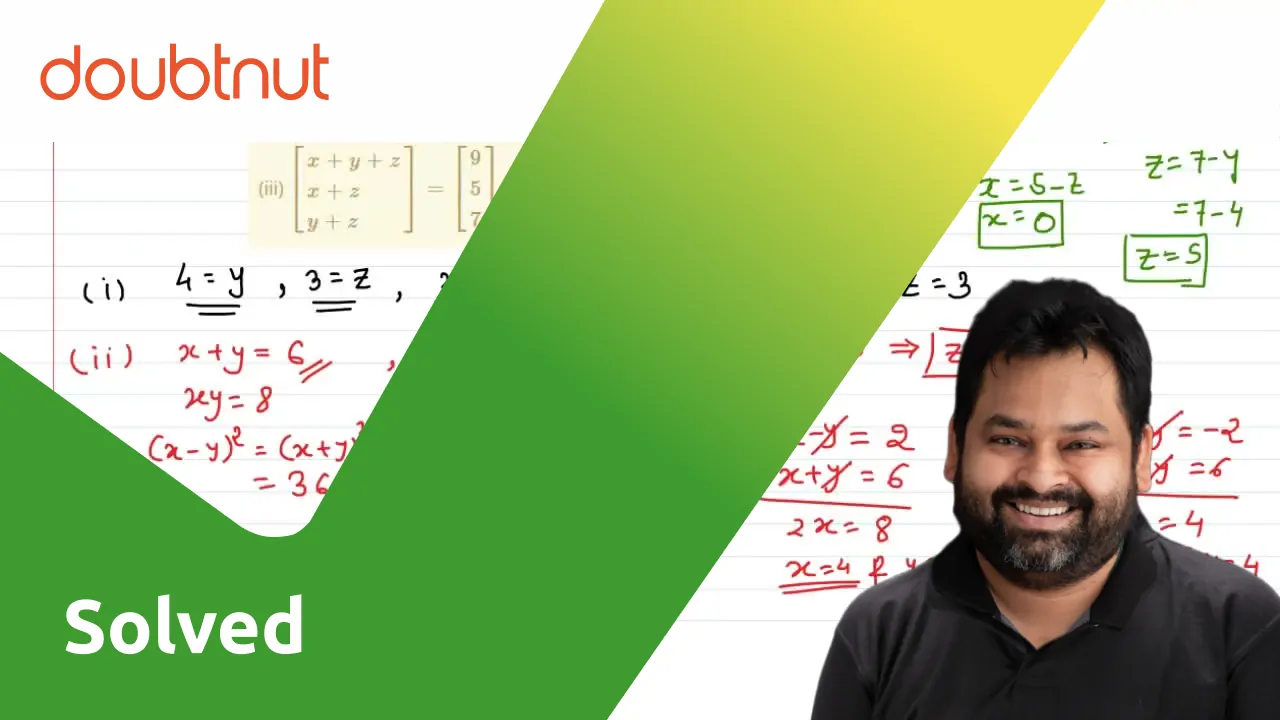Doubtnut App - Get 50 Lakh+ video solutions better than GoogleInstall Now

# Find the values of x, y and z from the following equations : (i) [43x5]=[yz15] (ii) [x+y25+zxy]= (iii) ⎡⎢⎣x+y+zx+zy+z⎤⎥⎦=⎡⎢⎣957⎤⎥⎦Solution in Gujrati

Text Solution
Verified by Experts

## The correct Answer is:(i) z=3. (iii) z=3

Video Solution